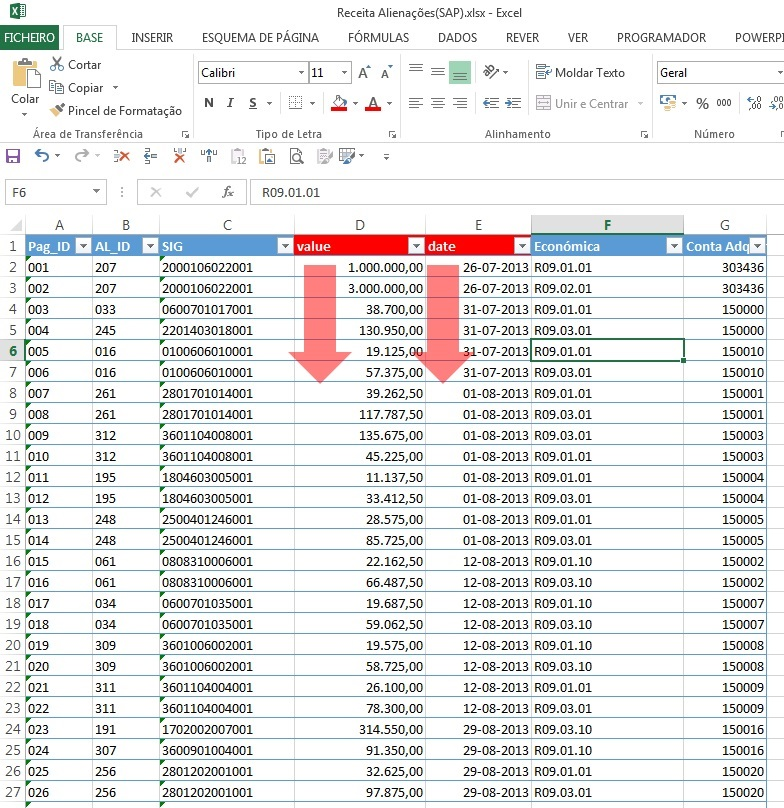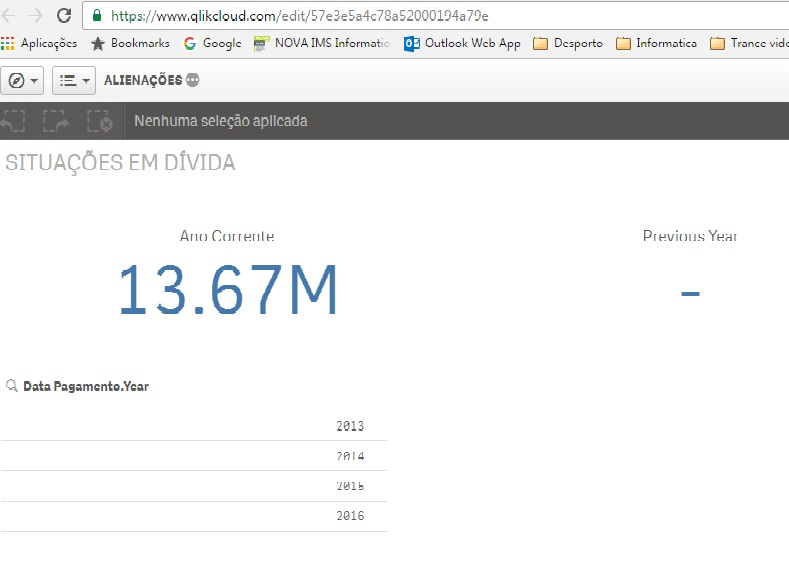# New to Qlik Sense

If you’re new to Qlik Sense, start with this Discussion Board and get up-to-speed quickly.

Announcements
QlikWorld 2023, a live, in-person thrill ride. Save \$300 before February 6: REGISTER NOW!
cancel
Showing results for
Did you mean:Not applicable

## KPI Returns value from current and previous YearSomeone can give me a formula for previous year sum value, as shown in the pictures?Current Year:

Sum({\$<[Date] = {">=\$(=Yearstart(Max([Date])))  <= \$(=Date(Max([Date])))"}>} value)

Previous Year: ?

2 RepliesPartner - Specialist

Try with: Sum({\$<[Date] = {">=\$(=Yearstart(Max([Date]),-1))  <= \$(=YearEnd(Max([Date]),-1))"}>} value)

EduardoMVP

Or this:

Sum({\$<[Date] = {">=\$(=YearStart(Max([Date]), -1))<=\$(=YearStart(Max([Date])) -1))"}>} value)Tags
Community Browser